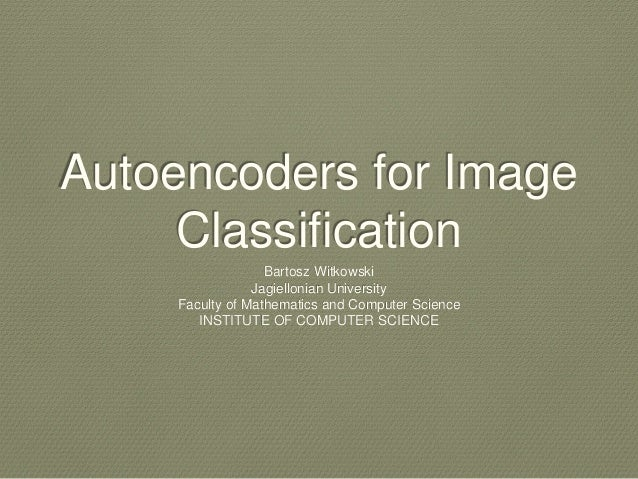Successfully reported this slideshow.Upcoming SlideShare
×

# Autoencoders for image_classification

4,321 views

Published on

Autoencoders for image classification,
Stacked Autoencoder and Stacked Convolutional Autoencoder

Published in: Software
• Full Name
Comment goes here.

Are you sure you want to Yes NoAre you sure you want to  Yes  No
• Dating direct: ❶❶❶ http://bit.ly/2F90ZZC ❶❶❶

Are you sure you want to  Yes  No

### Autoencoders for image_classification

1. 1. Autoencoders for Image Classification Bartosz Witkowski Jagiellonian University Faculty of Mathematics and Computer Science INSTITUTE OF COMPUTER SCIENCE
2. 2. Contents • Theoretical Background • Problem Formulation • Methodology • Results
3. 3. Theoretıcal Background • Artificial Neural Networks • Deep Neural Networks and Deep Learning • Autoencoders and Sparsity • Convolutional Networks
4. 4. artificial neural networks • The central idea is to extract linear combinations of the inputs as derived features and then model the target as a nonlinear function of these features • A feedforward neural network of depth n is a n- stage regression or classification model,
5. 5. The outputs of layer l are called activations and are computed based on linear combinations of inputs and the bias unit in the following way: Encoding Decoding
6. 6. soft-max activation function used as the last layer (classifier) for K-class classification Two types of activation functions: sigmoid activation and soft-max activation
7. 7. When training feedforward networks we use an average sum-of-squared errors as an error function To prevent from overfitting we add regularization to error function
8. 8. deep neural networks and deep learning • Deep vanilla neural networks perform worse than neural networks with one or two hidden layers. • In theory deep neural networks have at least the same expressive power as shallow neural networks but in practice they stuck in local optima during training phase. • It is important to use a non-linear activation function f(x) in each hidden layer
9. 9. autoencoders and sparsity • An autoencoder is a neural network that is trained to encode an input x into some representation c(x) so that the input can be reconstructed from that representation
10. 10. After successful3 training, it should decompose the inputs into a combination of hidden layer activations. With this trained autoencoder has learned features
11. 11. We can measure the average activations of the neurons in the second layer: and add a penalty to the error function which will prevent the activations from straying too far from some desired mean activation p (the sparsity parameter). * Kullback-Leibler divergence
12. 12. The resulting autoencoder is called a sparse autoencoder. B is called the sparsity constraint and controls the sparsity penalty.
13. 13. stacked autoencoders
14. 14. convolutional networks • Better than vanilla neural network. • Inspired by the human visual system structure and work by exploiting local connections through two operations ( Convolution and Sub- sampling / Pooling)
15. 15. convolution • Organized in layers of two types: • Convolution, Sub-sampling
16. 16. pooling • Biologically inspired operation that reduces the dimensionality of the input.
17. 17. Single cell of output matrix is calculated by: kernel, I is the input matrix. In actual implementation P
18. 18. problem Formulation • MNIST
19. 19. Dataset: Handwritten digits, Training Set: 60,000 examples Test Set: 10,000 examples Size: 28 x 28
20. 20. methodology • Architecture-1 Stacked Autoencoders • Artchitecture-2 Stacked Convolutional Autoencoders • Visualizing Features
21. 21. architecture-1 • 784-200-200-200-10 Deep network • Greedy layerwise training • Training protocol • Training Parameters and Methods
22. 22. greedy layer wise training • to construct a deep pretrained network of n layers divide the learning into n stages. • In the first stage train an autoencoder on the provided training data sans labels. • Next map the training data to the feature space. • The mapped data is then used to train the next stage auto encoder. • The training follows layer by layer until the last one. • The last layer is trained as a classifier (not as an autoencoder) using supervised learning.
23. 23. training protocol
24. 24. t: the first 30000 images (out of 60000
25. 25. After training the last stage, the networks n1 through n4 are stacked to form a deep neural network. Use the full training set to train the deep neural network – this final step is called fine-tuning.
26. 26. modify the weights W(1) as well, so that adjustments can be m
27. 27. archıtecture-2 • Instead of training the network on the full image we can exploit local connectivity via convolutional networks, and additionally restrict the number of trainable parameters with the use of pooling.
28. 28. visualizing features Activation of the hidden unit i
29. 29. Results
30. 30. difference of cnns and autoencoders • The main difference between AutoEncoder and Convolutional Network is the level of network hardwiring. Convolutional Nets are pretty much hardwired. Convolution operation is pretty much local in image domain, meaning much more sparsity in the number of connections in neural network view. Pooling(subsampling) operation in image domain is also a hardwired set of neural connections in neural domain. Such topological constraints on network structure. Given such constraints, training of CNN learns best weights for this convolution operation (In practice there are multiple filters). CNNs are usually used for image and speech tasks where convolutional constraints are a good assumption.
31. 31. • In contrast, Autoencoders almost specify nothing about the topology of the network. They are much more general. The idea is to find good neural transformation to reconstruct the input. They are composed of encoder (projects the input to hidden layer) and decoder (reprojects hidden layer to output). The hidden layer learns a set of latent features or latent factors. Linear autoencoders span the same subspace with PCA. Given a dataset, they learn number of basis to explain the underlying pattern of the data.
32. 32. Cenk Bircanoğlu “Thank You For Listening”